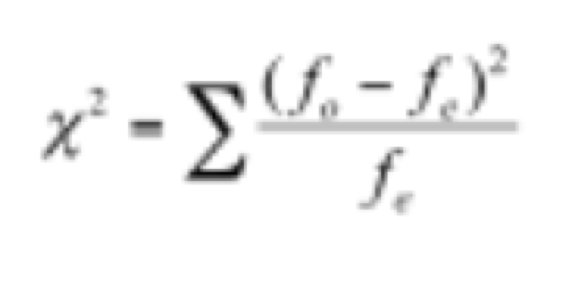# Chi-squared Distribution

## What is Chi-Square Distribution?

Chi Square distribution is the sum of the squares of independent normal random variables. This is most commonly used for testing relationships between categorical variables and creating confidence intervals. In a Chi-Square test, it is assumed that the categorical variables in a population are independent and no relationship exists between them.

Chi-Square is rather simplified and expressed as:fo = observed frequency (the observed counts in the cells)
fe = expected frequency if NO relationship existed between the variables

### How does the Chi-Square Distribution work?

In the most common usage, Chi-Square is used to run Tests of Independence with a bivariate table (crosstabulation). This table shows the distributions of two categorical variables at the same time, with the intersections of the category variables appearing in the table’s cells. The independence tests determine whether an association exists between the two variables by contrasting the observed pattern of responses in the cells to the expected pattern of truly independent variables.
Comparing the Chi-Square stats against a critical value from the Chi-Square distribution allows shows whether the observed cell counts are significantly higher or lower than the expected cell counts.